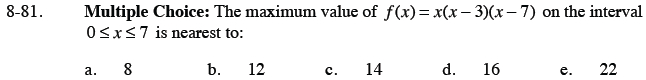### Home > CALC > Chapter 8 > Lesson 8.2.2 > Problem8-81

8-81.Expand f before differentiating.

Remember that a maximum value is a y-value, not an x-value.

Notice that this is a CLOSED domain: 0 ≤ x ≤ 7.
Since maxima can exist where f '(x) = 0 or where f '(x) = DNE, it is important to check the y-values at each endpoint.
f(0) = ________ and f(7) = ________
After all, an endpoint might exceed the y-values where f '(x) = 0; in which case, that endpoint will be in the global maximum.